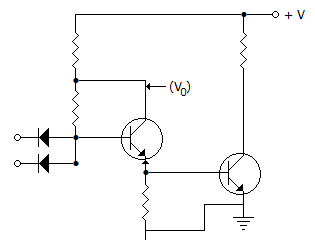# Electronics and Communication Engineering - Digital Electronics

41.

A 3 bit binary adder can add

 A. 2 bits B. 2 bits C. 3 bits D. 8 bits

Explanation:

No answer description available for this question. Let us discuss.

42.

The logic performed by the high-noise immunity logic circuit shown below isA. OR B. AND C. NOR D. NAND

Explanation:

No answer description available for this question. Let us discuss.

43.

An 8156 has A15 connected to its CE input. A14 to A8 are unconnected, and AD7 to AC0 are connected.Ignoring shadows, the port addresses

 A. 80 H B. 85 H C. 82 H, 83 H D. 80 H to 85 H

Explanation:

No answer description available for this question. Let us discuss.

44.

In 8085 microprocessor, what is the length of IR (instruction register)?

 A. 6 bits B. 8 bits C. 12 bits D. 16 bits

Explanation:

No answer description available for this question. Let us discuss.

45.

A sequence detector is required to give a logical output of 1 whenever the sequence 1011 is detected in the incoming pulse stream. Minimum number of flip-flops needed to built the sequence detector is

 A. 4 B. 3 C. 1 D. 2